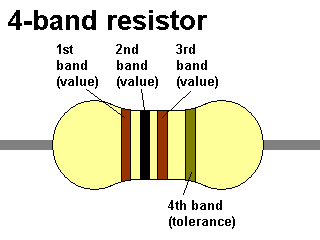# Component Calculators

## Resistor Colour Calculator:

This calculator can calculate the values of resistors using the standard 4 or 5 colour band system. The values are returned in ohms, k ohms and M ohms and a tolerance value is also given.

When reading resistors, you read from left to right in order - the right most band has a larger gap between itself and the rest of the bands on the left.1st Band:
2nd Band:
3rd Band:
Final Band:
Result (ohms):
Result (k):
Result (M):
Tolerance:

Notes:
With the 4 band resistor, the 4th band is selected using the last list of colours (this is the tolerance band).
Only Gold and Silver multiplier bands are supported with the five band resistor types by the 4th column.

## Capacitor Calculator

This calculator is simpler, just select the three numbers written on the capacitor and this calculator will return the value in pico farads, nano farads and micro farads.

1st Number:
2nd Number:
3rd Number: